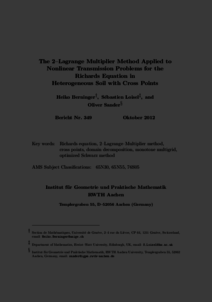Repository: Freie Universität Berlin, Math Department

# The 2-Lagrange multiplier method applied to nonlinear transmission problems for the Richards equation in heterogeneous soil with cross points

Berninger, H. and Loisel, S. and Sander, O. (2014) The 2-Lagrange multiplier method applied to nonlinear transmission problems for the Richards equation in heterogeneous soil with cross points. SIAM J. Sci. Comput., 36 (5). A2166-A2198. ISSN 1064-8275Preview

1MB

Official URL: https://dx.doi.org/10.1137/120901064

## Abstract

We formulate the 2-Lagrange multiplier method for the Richards equation in heterogeneous soil. This allows a rigorous formulation of a discrete version of the Richards equation on subdomain decompositions involving cross points. Using Kirchhoff transformation, the individual subdomain problems can be transformed to convex minimization problems and solved efficiently using a monotone multigrid method. We discuss and compare weak formulations of the time-discrete and fully discretized multi-domain problem. It is shown that in the case of two subdomains, when solving the resulting discrete system with a Richardson iteration, the new method is equivalent to the Robin method for the Richards equation proposed in . We give numerical results for a problem with realistic soil parameters.

Item Type: Article Mathematical and Computer Sciences > Mathematics > Numerical Analysis Department of Mathematics and Computer Science > Institute of Mathematics 1858 Ekaterina Engel 07 Mar 2016 14:33 03 Mar 2017 14:42

Repository Staff Only: item control page